Like   Tweet   Pin   +1   in
 /* styles */ Tai Chi Stories, Tips and Resources   ||   Issue #23 February 2020
 table div table+table+table div table{width:100%;padding:0}table div table+table+table div table img{width:96.23%;padding:0;float:none}table div table+table+table div table td{width:100%;padding:0 1.88% 18px}/* styles */Welcome to this year's first edition of Good Chi News. I hope you restored your Chi over the holidays and your new year has started well for you.

In this issue we have our usual inspirational Lao Tzu quote and a Tai Chi Tip on postural alignment. Plus two informative Youtube clips on relaxation and Tai Chi, and information on our two new services for 2020 - Sports Chi and our aged care Tai Chi Presentation

I hope you enjoy reading the issue.

Regards
Chris Bennett
Chris Chi

 table div table+table+table+table+table div table{width:100%;padding:0}table div table+table+table+table+table div table img{width:96.23%;padding:0;float:none}table div table+table+table+table+table div table td{width:100%;padding:0 1.88% 18px}/* styles */## Don't Keep Up - Keep On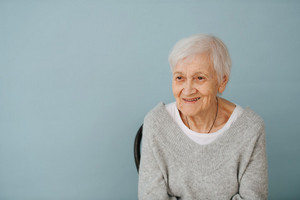One of the phrases I often repeat in Chair Chi sessions for residents is 'don't try to keep up - just keep on'.

I've found this eases the pressure to try and keep up with other residents, and it helps them to perform at their own pace and physical capabilities. Read More ...

 table div table+table+table+table+table+table+table+table div table{width:100%;padding:0}table div table+table+table+table+table+table+table+table div table img{width:96.23%;padding:0;float:none}table div table+table+table+table+table+table+table+table div table td{width:100%;padding:0 1.88% 18px}/* styles */## Tai Chi Sung (relaxation)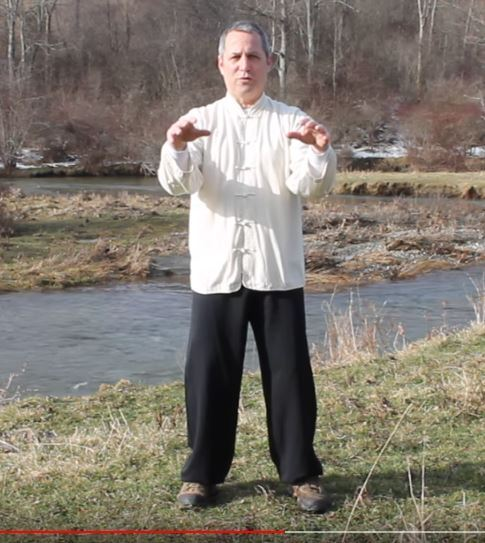'Rich Marantz of Green Mountain Tai Chi teaches an exercise to help understand the concept of Sung'.

A good and clear explanation and demonstration of the concept of sung.

As Rich Marantz expresses it, 'sung' is a 'relaxed aliveness' and the exercise he demonstrates is one that will help you achieve this.

 table div table+table+table+table+table+table+table+table+table+table+table div table{width:100%;padding:0}table div table+table+table+table+table+table+table+table+table+table+table div table img{width:96.23%;padding:0;float:none}table div table+table+table+table+table+table+table+table+table+table+table div table td{width:100%;padding:0 1.88% 18px}/* styles */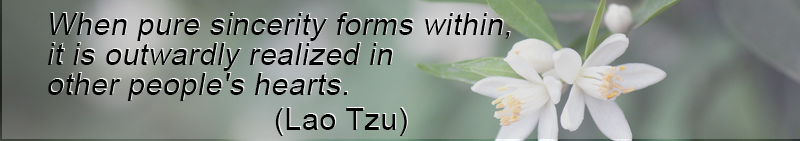table div table+table+table+table+table+table+table+table+table+table+table+table+table div table{width:100%;padding:0}table div table+table+table+table+table+table+table+table+table+table+table+table+table div table img{width:96.23%;padding:0;float:none}table div table+table+table+table+table+table+table+table+table+table+table+table+table div table td{width:100%;padding:0 1.88% 18px}/* styles */## Sports Chi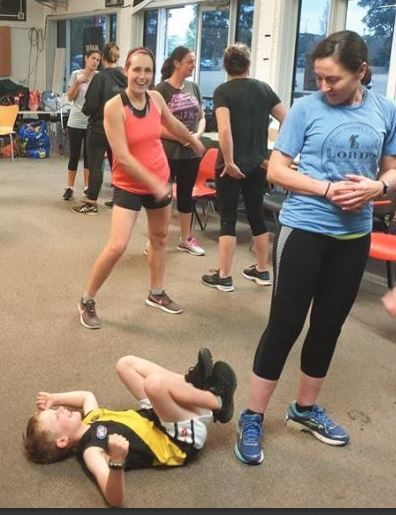Well, it's taken a while but I've finally ready to push ahead with my new Sports Chi program.

I've just had my flyer and logo professionally designed.

I finally get a chance to combine two passions - sport and Tai Chi.

Now for the billion phone calls required to get a workshop up and running :)

 table div table+table+table+table+table+table+table+table+table+table+table+table+table+table+table+table div table{width:100%;padding:0}table div table+table+table+table+table+table+table+table+table+table+table+table+table+table+table+table div table img{width:96.23%;padding:0;float:none}table div table+table+table+table+table+table+table+table+table+table+table+table+table+table+table+table div table td{width:100%;padding:0 1.88% 18px}/* styles */## Tai Chi Tip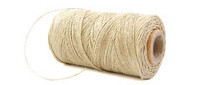Imagine a string attached to your head gently pulling you up to the sky. Knees are kept slightly bent.

 table div table+table+table+table+table+table+table+table+table+table+table+table+table+table+table+table+table+table+table div table{width:100%;padding:0}table div table+table+table+table+table+table+table+table+table+table+table+table+table+table+table+table+table+table+table div table img{width:96.23%;padding:0;float:none}table div table+table+table+table+table+table+table+table+table+table+table+table+table+table+table+table+table+table+table div table td{width:100%;padding:0 1.88% 18px}/* styles */## Tai Chi and Natural MovementI do like teacher Gene Burnett's views on Tai Chi and many of his clips are very informative.

Check out his reaction when he has to deal with an interruption (4.19) while recording this clip.

That's what I call good Tai Chi! :)

 table div table+table+table+table+table+table+table+table+table+table+table+table+table+table+table+table+table+table+table+table+table+table div table{width:100%;padding:0}table div table+table+table+table+table+table+table+table+table+table+table+table+table+table+table+table+table+table+table+table+table+table div table img{width:96.23%;padding:0;float:none}table div table+table+table+table+table+table+table+table+table+table+table+table+table+table+table+table+table+table+table+table+table+table div table td{width:100%;padding:0 1.88% 18px}/* styles */## Tai Chi Presentation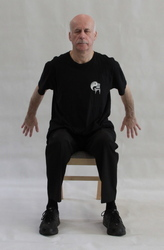I've been busy promoting our local Tai Chi presentation for aged care residents and have several bookings so far.

If you are in the Northern, Southern and Eastern parts of Melbourne and interested in booking a session for your residents, give me a call.

 table div table+table+table+table+table+table+table+table+table+table+table+table+table+table+table+table+table+table+table+table+table+table+table+table+table div table{width:100%;padding:0}table div table+table+table+table+table+table+table+table+table+table+table+table+table+table+table+table+table+table+table+table+table+table+table+table+table div table img{width:96.23%;padding:0;float:none}table div table+table+table+table+table+table+table+table+table+table+table+table+table+table+table+table+table+table+table+table+table+table+table+table+table div table td{width:100%;padding:0 1.88% 18px}/* styles */table div table+table+table+table+table+table+table+table+table+table+table+table+table+table+table+table+table+table+table+table+table+table+table+table+table+table+table div table{width:100%;padding:0}table div table+table+table+table+table+table+table+table+table+table+table+table+table+table+table+table+table+table+table+table+table+table+table+table+table+table+table div table img{width:96.23%;padding:0;float:none}table div table+table+table+table+table+table+table+table+table+table+table+table+table+table+table+table+table+table+table+table+table+table+table+table+table+table+table div table td{width:100%;padding:0 1.88% 18px}/* styles */